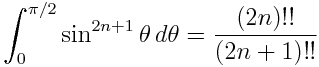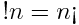# Variations on factorial!

If you’ve heard of factorial, have you heard of double factorial or subfactorial?

Double factorial is written n!!. The factorial of a positive integer n is the product of all positive integers less than or equal to n. The double factorial of n is the product of all integers less than or equal to n that have the same parity.  That is, for an odd number n,  the product defining n!! includes only odd integers and for an even integer n, the product defining n!! includes only even integers. For example, 7!! = 7 × 5 × 3 × 1 and 8!! = 8 × 6 × 4 × 2. By definition, 0!! and -1!! equal 1.

Double factorials often arise in integrals and power series and make it possible to state equations succinctly that would be verbose otherwise. For example,It’s possible to define higher factorials or multifactorials. For instance n!!!, the triple factorial of n, is the product of positive integers less than or equal to n and congruent to n mod 3. So, for example, 8!!! = 8 × 5 × 2.

Factorials count the number of ways a set can be arranged. A set with n distinct elements can be arranged in n! ways. The number of arrangements that move every element from its original position is the subfactorial of n. Sometimes subfactorial is written with the exclamation point in front of its argument and sometimes it is written with an inverted exclamation point following its argument, i.e.(By the way, the inverted exclamation mark, used to mark the beginning of an exclamatory sentence in Spanish, is Unicode character U+00A1. You can produce it in HTML with &iexl;. In TeX, you can produce it ! outside of math mode and mbox{!} in math mode.)

Subfactorial can be computed from the factorial byfor positive n where ⌊x⌋ is the greatest integer less than x. The subfactorial of 0 is defined to be 1.

Update: See post on mutifactorials.

## Related posts## 13 thoughts on “Variations on factorial!”

1. I didn’t know that simple expression for the double factorial. Will keep it in mind (although I am not really expanding that many integrals these days, I used to do it 2 years ago).

Cheers,

Ruben@mostlymaths.net

2. Is there such a thing as a prime factorial? That would be the product of all prime numbers less than or equal to n. Would such a thing have any utility?

3. @christopher: surely the Riemann hypothesis folks could find use for such a thing :)

5. Ooops, primorial, not radical (the radical only includes primes dividing n). Thanks Nathaniel!

6. Hendree Milward

I have been thinking about a generalization of factorials along the lines of operation. So the normal factorial would be the multiplicative factorial. The additive factorial of n would be sum of the first n integers. The exponential factorial would be n^n-1^n-2^….. It’s a neat way to turn any binary opp on the naturals into a monary opp. Any ideas what these are called?

7. This is related to map-reduce in programming. Summing and multiplying are two common ways of “reducing” an operation mapped onto a sequence.

8. Double factorial can be expressed with the Gamma-function too, just as (single) factorial (Arfken 1985, p. 548). This quite surpized me.

9. I would have thought : n!!=(n!)!

10. Is there a combinatorial interpretation for the multifactorial?

11. That’s a very good question. They come up so often that there must be a combinatorial interpretation, but I can’t think of one off hand.

12. Minor correction:
“For instance n!!!, the triple factorial of n, is the product of positive integers less than n and congruent to n mod 3. So, for example, 8!!! = 8 × 5 × 2.”
Should be:
“For instance n!!!, the triple factorial of n, is the product of positive integers less than or equal to n and congruent to n mod 3. So, for example, 8!!! = 8 × 5 × 2.”